# Work and energy part a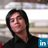Physics Specialist / Project Engineer at Renewable Energy Technology Center à University of Santo Tomas, Manila
27 May 2021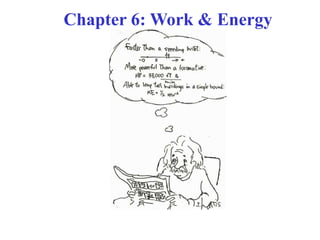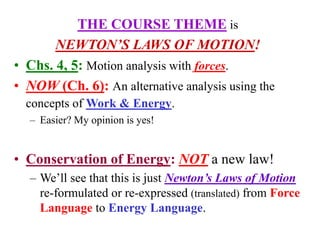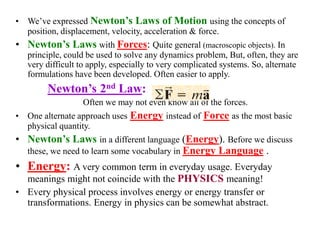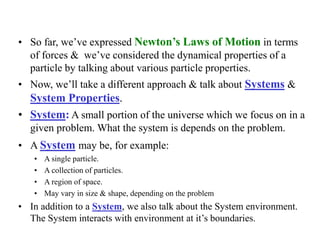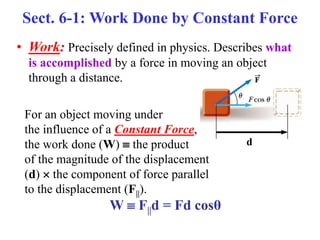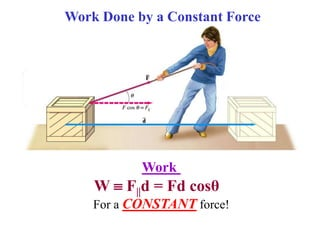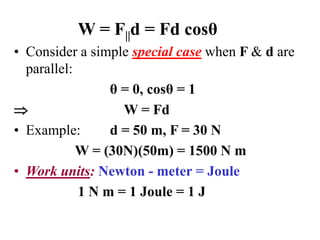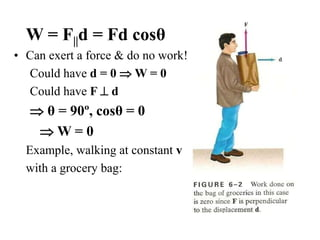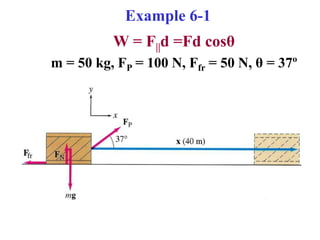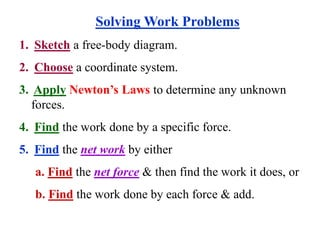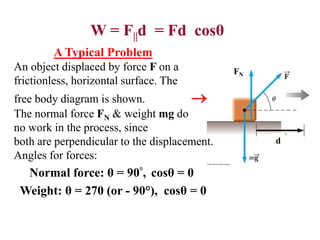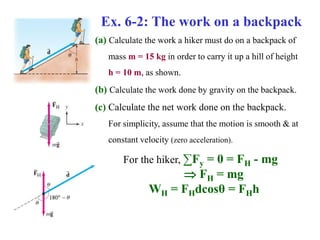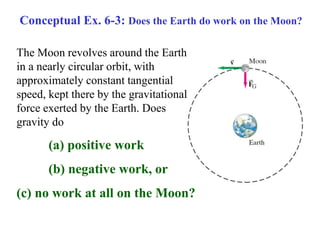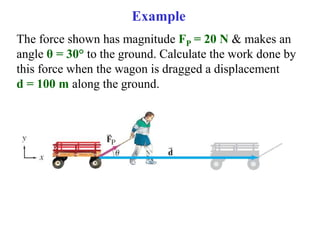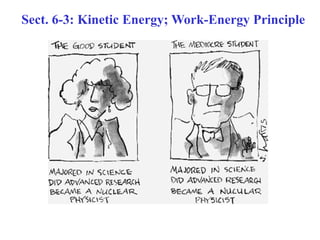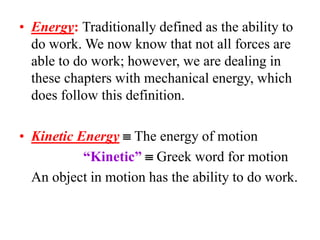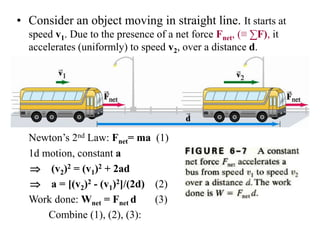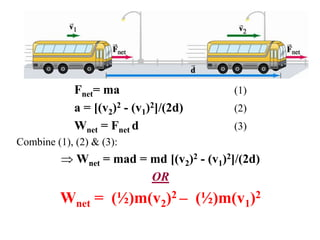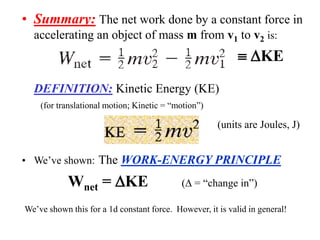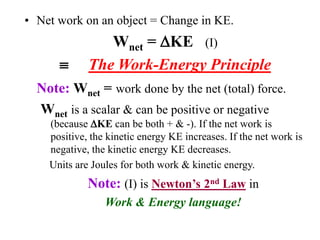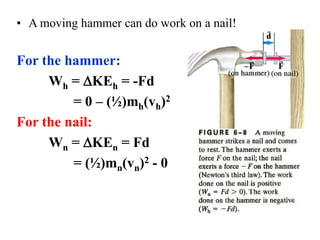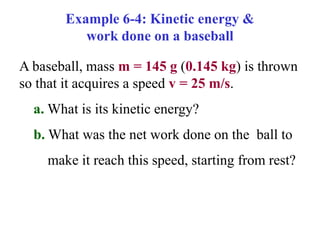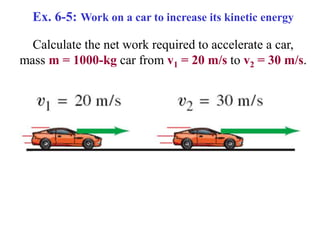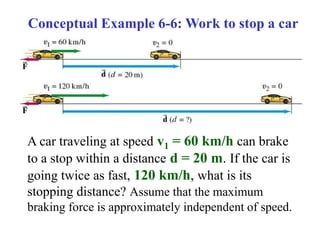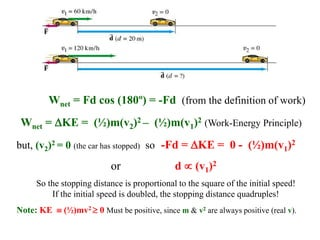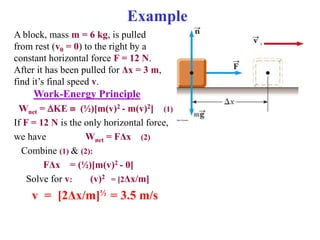1 sur 26

### Work and energy part a

• 1. Chapter 6: Work & Energy
• 2. THE COURSE THEME is NEWTON’S LAWS OF MOTION! • Chs. 4, 5: Motion analysis with forces. • NOW (Ch. 6): An alternative analysis using the concepts of Work & Energy. – Easier? My opinion is yes! • Conservation of Energy: NOT a new law! – We’ll see that this is just Newton’s Laws of Motion re-formulated or re-expressed (translated) from Force Language to Energy Language.
• 3. • We’ve expressed Newton’s Laws of Motion using the concepts of position, displacement, velocity, acceleration & force. • Newton’s Laws with Forces: Quite general (macroscopic objects). In principle, could be used to solve any dynamics problem, But, often, they are very difficult to apply, especially to very complicated systems. So, alternate formulations have been developed. Often easier to apply. Newton’s 2nd Law: Often we may not even know all of the forces. • One alternate approach uses Energy instead of Force as the most basic physical quantity. • Newton’s Laws in a different language (Energy). Before we discuss these, we need to learn some vocabulary in Energy Language . • Energy: A very common term in everyday usage. Everyday meanings might not coincide with the PHYSICS meaning! • Every physical process involves energy or energy transfer or transformations. Energy in physics can be somewhat abstract.
• 4. • So far, we’ve expressed Newton’s Laws of Motion in terms of forces & we’ve considered the dynamical properties of a particle by talking about various particle properties. • Now, we’ll take a different approach & talk about Systems & System Properties. • System: A small portion of the universe which we focus on in a given problem. What the system is depends on the problem. • A System may be, for example: • A single particle. • A collection of particles. • A region of space. • May vary in size & shape, depending on the problem • In addition to a System, we also talk about the System environment. The System interacts with environment at it’s boundaries.
• 5. Sect. 6-1: Work Done by Constant Force • Work: Precisely defined in physics. Describes what is accomplished by a force in moving an object through a distance. For an object moving under the influence of a Constant Force, the work done (W)  the product of the magnitude of the displacement (d)  the component of force parallel to the displacement (F||). W  F||d = Fd cosθ d
• 6. Work Done by a Constant Force Work W  F||d = Fd cosθ For a CONSTANT force!
• 7. W = F||d = Fd cosθ • Consider a simple special case when F & d are parallel: θ = 0, cosθ = 1  W = Fd • Example: d = 50 m, F = 30 N W = (30N)(50m) = 1500 N m • Work units: Newton - meter = Joule 1 N m = 1 Joule = 1 J
• 8. W = F||d = Fd cosθ • Can exert a force & do no work! Could have d = 0  W = 0 Could have F  d  θ = 90º, cosθ = 0  W = 0 Example, walking at constant v with a grocery bag:
• 9. Example 6-1 W = F||d =Fd cosθ m = 50 kg, FP = 100 N, Ffr = 50 N, θ = 37º
• 10. Solving Work Problems 1. Sketch a free-body diagram. 2. Choose a coordinate system. 3. Apply Newton’s Laws to determine any unknown forces. 4. Find the work done by a specific force. 5. Find the net work by either a. Find the net force & then find the work it does, or b. Find the work done by each force & add.
• 11. W = F||d = Fd cosθ A Typical Problem An object displaced by force F on a frictionless, horizontal surface. The free body diagram is shown.  The normal force FN & weight mg do no work in the process, since both are perpendicular to the displacement. Angles for forces: Normal force: θ = 90°, cosθ = 0 Weight: θ = 270 (or - 90°), cosθ = 0 d FN
• 12. Ex. 6-2: The work on a backpack For the hiker, ∑Fy = 0 = FH - mg  FH = mg WH = FHdcosθ = FHh (a) Calculate the work a hiker must do on a backpack of mass m = 15 kg in order to carry it up a hill of height h = 10 m, as shown. (b) Calculate the work done by gravity on the backpack. (c) Calculate the net work done on the backpack. For simplicity, assume that the motion is smooth & at constant velocity (zero acceleration).
• 13. Conceptual Ex. 6-3: Does the Earth do work on the Moon? The Moon revolves around the Earth in a nearly circular orbit, with approximately constant tangential speed, kept there by the gravitational force exerted by the Earth. Does gravity do (a) positive work (b) negative work, or (c) no work at all on the Moon?
• 14. The force shown has magnitude FP = 20 N & makes an angle θ = 30° to the ground. Calculate the work done by this force when the wagon is dragged a displacement d = 100 m along the ground. Example
• 15. Sect. 6-3: Kinetic Energy; Work-Energy Principle
• 16. • Energy: Traditionally defined as the ability to do work. We now know that not all forces are able to do work; however, we are dealing in these chapters with mechanical energy, which does follow this definition. • Kinetic Energy  The energy of motion “Kinetic”  Greek word for motion An object in motion has the ability to do work.
• 17. • Consider an object moving in straight line. It starts at speed v1. Due to the presence of a net force Fnet, (≡ ∑F), it accelerates (uniformly) to speed v2, over a distance d. Newton’s 2nd Law: Fnet= ma (1) 1d motion, constant a  (v2)2 = (v1)2 + 2ad  a = [(v2)2 - (v1)2]/(2d) (2) Work done: Wnet = Fnet d (3) Combine (1), (2), (3):
• 18. Fnet= ma (1) a = [(v2)2 - (v1)2]/(2d) (2) Wnet = Fnet d (3) Combine (1), (2) & (3):  Wnet = mad = md [(v2)2 - (v1)2]/(2d) OR Wnet = (½)m(v2)2 – (½)m(v1)2
• 19. • Summary: The net work done by a constant force in accelerating an object of mass m from v1 to v2 is:  KE DEFINITION: Kinetic Energy (KE) (for translational motion; Kinetic = “motion”) (units are Joules, J) • We’ve shown: The WORK-ENERGY PRINCIPLE Wnet = KE ( = “change in”) We’ve shown this for a 1d constant force. However, it is valid in general!
• 20. • Net work on an object = Change in KE. Wnet = KE (I)  The Work-Energy Principle Note: Wnet = work done by the net (total) force. Wnet is a scalar & can be positive or negative (because KE can be both + & -). If the net work is positive, the kinetic energy KE increases. If the net work is negative, the kinetic energy KE decreases. Units are Joules for both work & kinetic energy. Note: (I) is Newton’s 2nd Law in Work & Energy language!
• 21. • A moving hammer can do work on a nail! For the hammer: Wh = KEh = -Fd = 0 – (½)mh(vh)2 For the nail: Wn = KEn = Fd = (½)mn(vn)2 - 0
• 22. Example 6-4: Kinetic energy & work done on a baseball A baseball, mass m = 145 g (0.145 kg) is thrown so that it acquires a speed v = 25 m/s. a. What is its kinetic energy? b. What was the net work done on the ball to make it reach this speed, starting from rest?
• 23. Ex. 6-5: Work on a car to increase its kinetic energy Calculate the net work required to accelerate a car, mass m = 1000-kg car from v1 = 20 m/s to v2 = 30 m/s.
• 24. Conceptual Example 6-6: Work to stop a car A car traveling at speed v1 = 60 km/h can brake to a stop within a distance d = 20 m. If the car is going twice as fast, 120 km/h, what is its stopping distance? Assume that the maximum braking force is approximately independent of speed.
• 25. Wnet = Fd cos (180º) = -Fd (from the definition of work) Wnet = KE = (½)m(v2)2 – (½)m(v1)2 (Work-Energy Principle) but, (v2)2 = 0 (the car has stopped) so -Fd = KE = 0 - (½)m(v1)2 or d  (v1)2 So the stopping distance is proportional to the square of the initial speed! If the initial speed is doubled, the stopping distance quadruples! Note: KE  (½)mv2  0 Must be positive, since m & v2 are always positive (real v).
• 26. Example A block, mass m = 6 kg, is pulled from rest (v0 = 0) to the right by a constant horizontal force F = 12 N. After it has been pulled for Δx = 3 m, find it’s final speed v. Work-Energy Principle Wnet = KE  (½)[m(v)2 - m(v)2] (1) If F = 12 N is the only horizontal force, we have Wnet = FΔx (2) Combine (1) & (2): FΔx = (½)[m(v)2 - 0] Solve for v: (v)2 = [2Δx/m] v = [2Δx/m]½ = 3.5 m/s l l